Latest Banking jobs   »   Quantitative Aptitude Quiz For FCI Phase...

# Quantitative Aptitude Quiz For FCI Phase I 2022- 20th December

Q1. Find the probability of selecting two red cards from a deck of 52 cards?

(a) 37/102
(b) 25/102
(c) 19/102
(d) 14/51
(e) None of these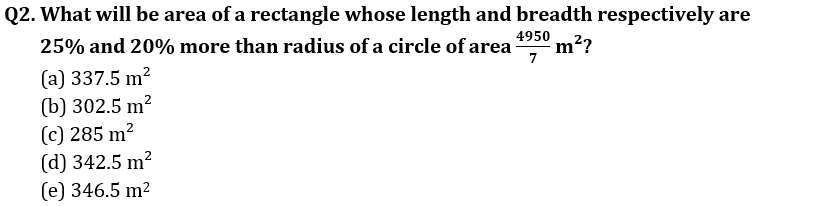Q3. Income of B is 40% more than income of A, find how much percent income of B is reduced so that it becomes equal to 105% of Income of A?
(a) 15%
(b) 20%
(c) 25%
(d) 10%
(e) can’t be determine.

Q4. A, B and C started a business by investing Rs 45000, Rs 55000 and Rs 65000 respectively. A is working partner and gets 12% of the profit as working allowance and remaining profit is distributed in the proportion of their investment. If money received by C (in end of a year) is Rs 23400. Find total profit?
(a) Rs 62500
(b) Rs 57500
(c) Rs 60000
(d) Rs 67500
(e) None of these

Q5. If a train runs with 63 km/h it reaches, it’s destination 8 min earlier and if it runs with 49 km/h it become late by 12 min. Find total distance?
(a) 72 km
(b) 70.5 km
(c) 71 km
(d) 72.5km
(e) 73.5 km

Q6. If distance between A and B is 800 km. A man travelling from A at 40 km/hr started at 8 am and another man travelling from B at 50 km/hr at 10 am, then at what time, they will cross each other?
(a) 5 pm
(b) 4 pm
(c) 6 pm
(d) 7 pm
(e) 8 pm

Q7. If ratio between ages of A and B is in ratio 6 : 8 and between ages of B and C is 5 : 4 and if difference between ages of A and B is 10 yrs, what will be ages of C after 4 yrs?
(a) 32 yr
(b) 36 yr
(c) 40 yr
(d) 35 yr
(e) None of these

Q8. In a mixture of 50 litre, there is 20 litre water in it. What amount of water will be added to make water solution 60% in the mixture?
(a) 25 litre
(b) 20 litre
(c) 30 litre
(d) 28 litre
(e) None of these

Q9. If length of a rectangle is equal the radius of circle, whose area is 616 cm² and its breadth is 10 cm, then find area of rectangle?
(a) 150 cm²
(b) 180 cm²
(c) 140 cm²
(d) 160 cm²
(e) 170 cm²

Q10. If A, B and C completed a work in 12, 15 and 20 days respectively, and total amount received by them for the work is 360 Rs, then amount received by C is?
(a) 140 Rs.
(b) 180 Rs.
(c) 120 Rs.
(d) 90 Rs.
(e) None of these

Q11. If a rectangular iron slab of length, breadth and thickness of 24 cm, 18 cm and 4 cm respectively is melted to form a cube, then find the total surface area of the cube.
(a) 468 cm²
(b) 578 cm²
(c) 748 cm²
(d) 864 cm²
(e) 684 cm²

Q12. Dharam and Ankit starts a journey from same place. Ankit reached the destination 20 min before Dharam. If the speed of Ankit and Dharam is 90 kmph and 80 kmph. Find the distance cover by them.
(a) 220 km
(b) 260 km
(c) 250 km
(d) 320 km
(e) 240 km

Q13. Time taken by three persons A, B and C to complete a work alone are in the ratio of 3 : 4 : 5. If C works with 25% more efficient than his usual efficiency with B, then they complete the work in 8 days. Find time taken by A to complete the work alone.
(a) 15 days
(b) 20 days
(c) 18 days
(d) 12 days
(e) 10 days

Q14. Shivam has certain money. He spends 20% on insurance, invests 15% in share market, 35% on simple interest at rate of 20% per annum and saves remaining Rs 1260. Find interest earned by Shivam after 1 year?
(a) Rs 336
(b) Rs 294
(c) Rs 168
(d) Rs 236
(e) Rs 184

Q15. Deepak started a business with an investment of Rs 36000. After few months Harish joined him with an investment of Rs 45000. If at the end of the year, they share the profit in the ratio (Harish : Deepak) is 15 : 16, then find after how much time Harish joined business?
(a) 3 months
(b) 7 months
(c) 5 months
(d) 9 months
(e) 6 months

Solutions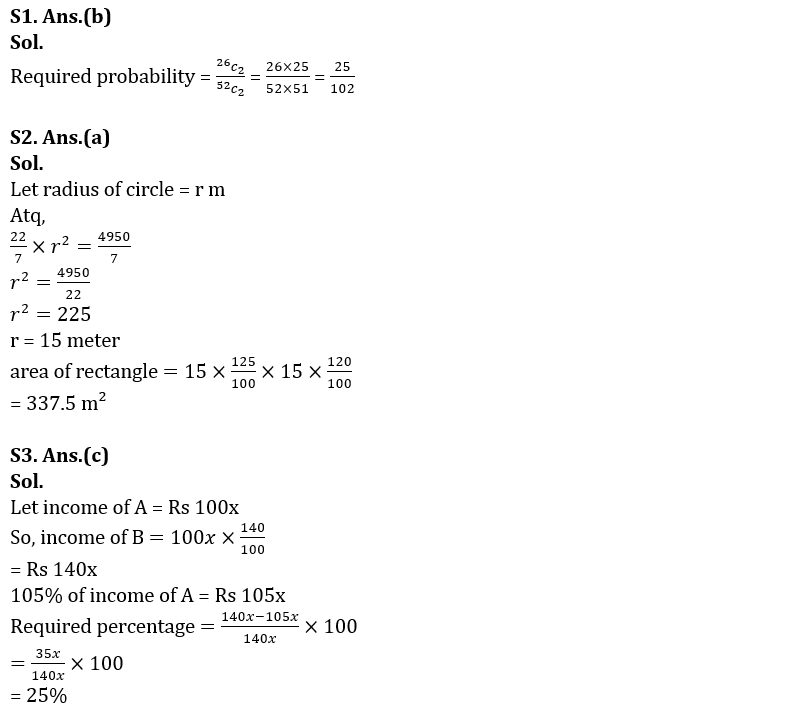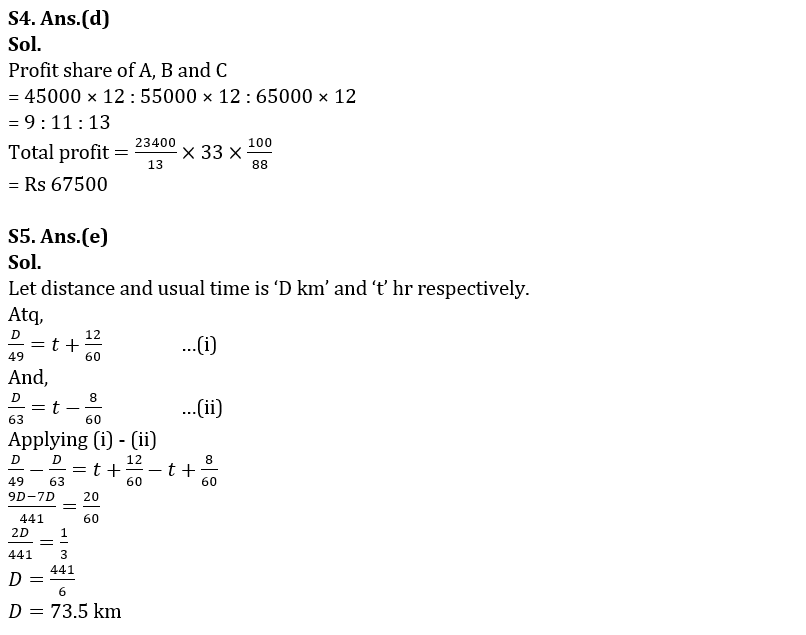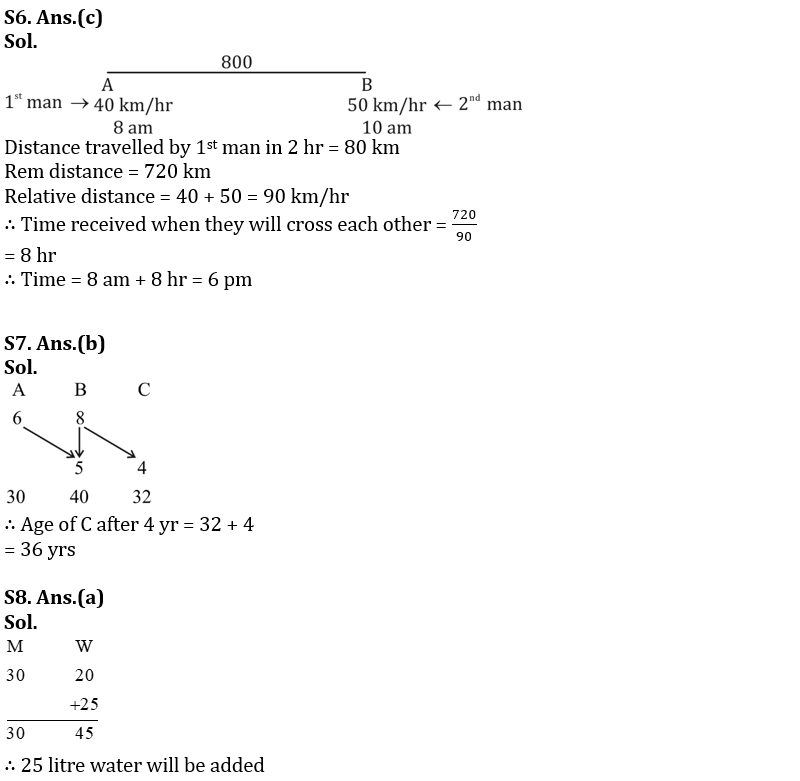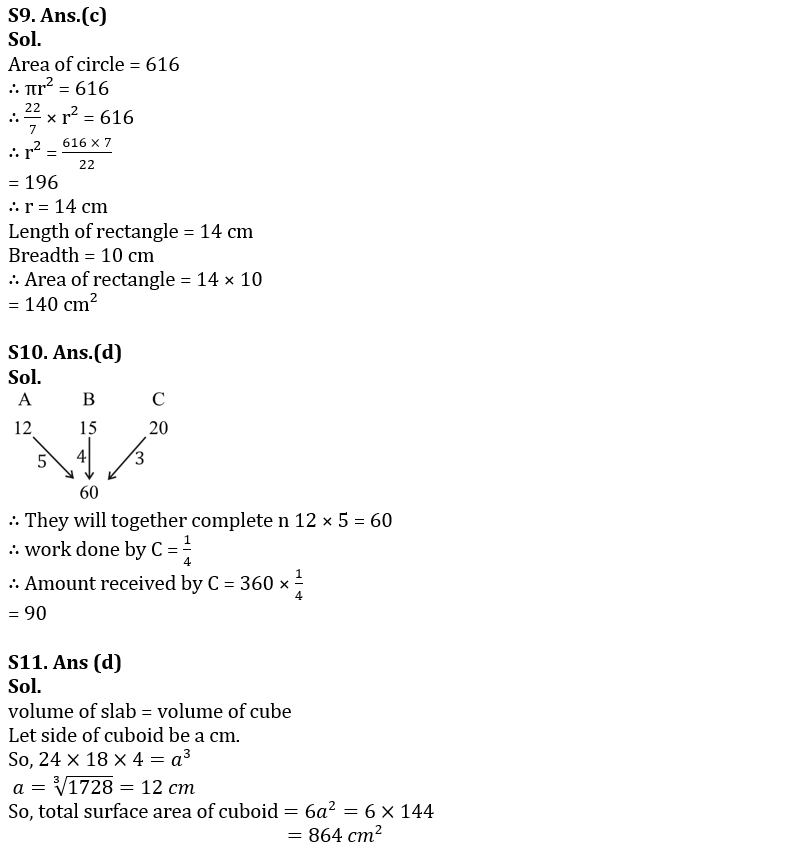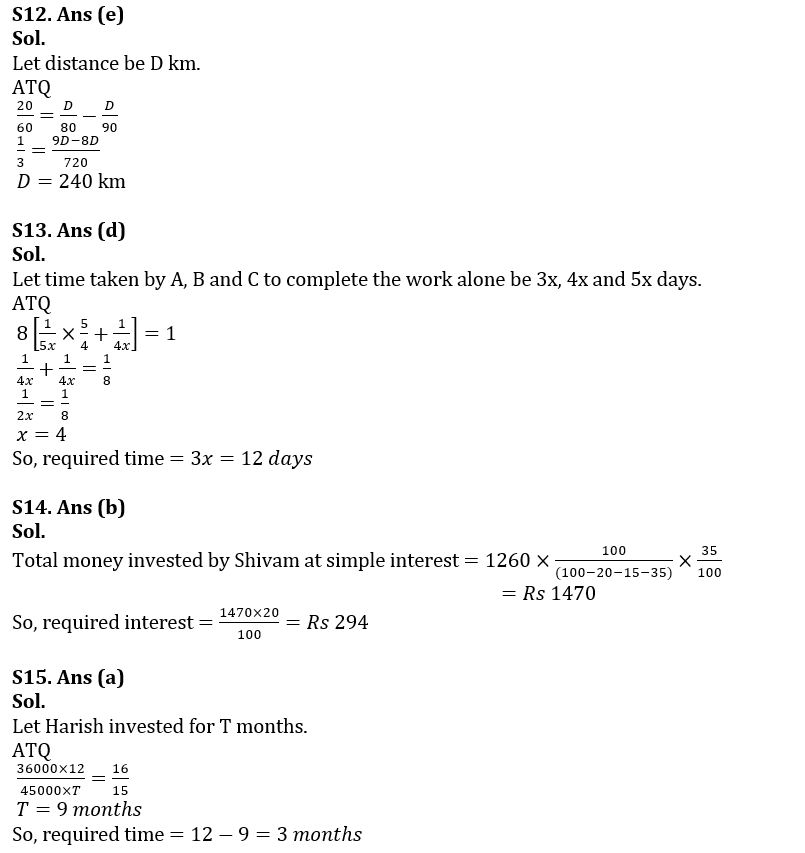#### Congratulations!Union Budget 2023-24: Free PDF